• 二项式似然函数
千次阅读 多人点赞
2019-04-03 19:52:12

# 1. 似然函数与概率

## 1.1 似然函数与概率的初步认识

似然函数，在机器学习的算法模型中，可以说是“老熟人”了，虽然总感觉自己已经掌握了这个概念，但是每次遇到具体的情况时，发现还是难以说清楚，于是根据wiki上关于Likelihood function的解释，以及个人的学习理解，整理笔记如下：

在统计学中，似然函数（或简称似然）是一个基于给定数据模型参数的特殊函数，似然(likelihood)与概率(probability)所表示的意义是完全不同的，具体如下：

给定参数值的情况下，概率用于描述未来出现某种情况的观测数据的可信度。
给定观测数据的情况下，似然用于描述参数值的可信度。

听起来可能会觉得摸不着头脑，先不着急，待我给出似然的定义之后，我们再来结合具体实例来理解上面的两句话。

## 1.2 似然的定义

根据概率的分布属于离散型还是连续型，似然函数的定义也略有不同，下面分别进行说明：

（1）对于离散型变量

假设X是一个离散型的随机变量，它的概率分布p取决于参数θ，那么它的似然函数定义为：

L ( θ ∣ x ) = p θ ( x ) = P θ ( X = x ) = P ( X = x ∣ θ ) = P ( X = x ; θ ) L(\theta | x)=p_{\theta}(x)=P_{\theta}(X=x)=P(X=x | \theta)=P(X=x ; \theta)       【】其中 L ( θ ∣ x ) L(\theta | x) L ( Θ = θ ∣ X = x ) L(\Theta=\theta | X=x) 的缩写，它表示基于给定的X=x，我们认为参数Θ=θ的似然（可信度），它的值则等于基于给定的参数Θ=θ，我们预测出现X=x的概率（可信度）（概率有好几种表达方式，这里全部列举了出来…

（2）对于连续型变量

假设X是一个连续型的随机变量，它的概率分布是一个概率密度函数ff取决于参数θ），这时它的似然函数定义为：
L ( θ ∣ x ) = f θ ( x ) {L}(\theta |{x})={f}_{\theta}({x})       【 L ( θ ∣ x ) L(\theta | x) 的含义与上面一致，不做解释, f θ ( x ) {f}_{\theta}({x}) 表示基于参数 θ ，预计 X=x 的概率值为 f θ ( x ) {f}_{\theta}({x}) ，也可写作 f ( x ∣ θ ) {f}({x} | \theta) 。这个看着有点抽象，举个具体实例来进行说明。
比如说X服从正态分布，我们知道正态分布的概率密度函数形式是：
f ( x ) = 1 σ 2 π e − ( x − μ ) 2 2 σ 2 f(x)=\frac{1}{\sigma \sqrt{2 \pi}} e^{-\frac{(x-\mu)^{2}}{2 \sigma^{2}}}        在机器学习的模型中，我们假设误差 ε 是独立同分布的，并且服从N(μ,σ2) 的高斯/正太分布，即ε相当于上面概率密度函数中的x。在线性回归模型中，我们知道 y(i)T· x(i) + ε(i) ，其中x(i)y(i) 表示是第i个样本的特征向量和真实标签值。

于是代入 ε(i) = y(i) - θT· x(i) 到正太分布的概率密度函数中，我们可以得到，对于已经观测到的m个样本的结果，它的似然函数为：

L ( θ ) = ∏ i = 1 m p ( y ( i ) ∣ x ( i ) ; θ ) = ∏ i = 1 m 1 2 π σ exp ⁡ ( − ( y ( i ) − θ T x ( i ) − μ ) 2 2 σ 2 ) L(\theta)=\prod_{i=1}^{m} p\left(y^{(i)} | x^{(i)} ; \theta\right) =\prod_{i=1}^{m} \frac{1}{\sqrt{2 \pi} \sigma} \exp \left(-\frac{\left(y^{(i)}-\theta^{T} x^{(i)}-\mu\right)^{2}}{2 \sigma^{2}}\right)       【】我们已经观测到m个样本的结果，每个样本之间是独立同分布的，于是计算m个样本同时发生的概率，则将每个样本发生的概率相乘。

这里讲述关于正态分布的例子，是我本文写完后加的，主要是希望有助于理解连续型变量的概率与似然之间的联系，如果不好理解的话，建议先阅读完下面的掷硬币例子，虽然是一个离散型变量的案例，但二者的原理是很相似的。

## 1.3 结合具体实例来深入理解似然与概率

在了解完似然函数的定义之后，我们接下来通过实例来理解最上面灰色框中的那两句话。

考虑经典的掷硬币问题，定义参数ρ1表示硬币的公平性。这个参数ρ1即为掷硬币时正面朝上的概率，ρ1的取值可以为 [0, 1] 区间的任意一个值。当然，对于一个均匀的硬币来说，ρ1的取值应该是0.5

现在假设我们掷了两次硬币，假设每次掷硬币的结果是独立同分布的(简称i.i.d.)，那么在给定参数值ρ1=0.5的情况下，预计观测到两次正面朝上的概率为：

P( 两次正面朝上 | ρ1=0.5) = 0.52 = 0.25

于是，根据前面关于离散型随机变量，似然函数的定义，我们知道，基于给定的观测数据（观测到两次正面朝上），我们认为参数ρ1=0.5似然(likelihood)为0.25，数学式写作：

L(ρ1=0.5 | 两次正面朝上) = 0.25

注意哦，这里可不能按照概率表达式的理解，读作在两次正面朝上的情况下，ρ1=0.5的概率为0.25。它实际上表达的含义是，在观测到两次正面朝上的情况下，我们推测硬币正面朝上的概率ρ1=0.5的可信度为0.25。

现在假设这是一枚不均匀的硬币，正面朝上的概率ρ1=0.3，在这个情况下，连续两次正面朝上的概率为：

P( 两次正面朝上 | ρ1=0.3) = 0.32 = 0.09

同理，基于观测数据（两次正面朝上），我们认为参数ρ1=0.3似然(likelihood)为0.09，数学式写作：

L(ρ1=0.3 | 两次正面朝上) = 0.09

翻译一下上式的含义，在观测到两次正面朝上的情况下，我们推测硬币正面朝上的概率ρ1=0.3的可信度为0.09。

读到这里的时候，是不是感觉好像有点尔理解了似然的意义了！它更加符合我们的主观认识，如果我们连续掷硬币两次，两次结果都是正面朝上，那么肯定会认为硬币正面朝上的概率为0.5的可信度比硬币正面朝上的概率为0.3的可信度高吧！

那么现在，我希望你能够理解上面的这两句话了。

给定参数值的情况下，概率用于描述未来出现某种情况的观测数据的可信度。
给定观测数据的情况下，似然用于描述参数值的可信度。

# 2. 极大似然估计

理解了概率似然的区别与联系之后，我们趁热打铁，赶紧来学习一下另外一个很常见的概念极大似然估计(MLE)！

一句话来定义极大似然估计如下：

在给定观测数据的情况下，某个参数值有多个取值可能，但是如果存在某个参数值，使其对应的似然值最大，那就说明这个值就是该参数最可信的参数值

我们极大似然估计的目的，就是为了找到似然最大时所对应的参数，这个方法在机器学习中经常用到！

仍然用前面掷硬币的例子来进行说明，假设我们连续掷一枚硬币两次，观测到的结果是两次正面朝上，我们要求硬币正面朝上的概率ρ1极大似然估计，过程如下：

假设硬币出现正面朝上的概率ρ1的值为θ，那么似然函数为：

L(ρ1=θ | 两次正面朝上) = θ2       其中θ∈[0, 1]

接下来就是简单的数学问题了，给定函数f(x) = x2x∈[0, 1]f(x) 的最大值。一个简单的思路，就是求一阶导，然后分析自变量在给定区间的单调性，然后找出函数的最大值，以及对应的x

于是在本例中，我们回想一下二次函数的图像就知道，L(ρ1=θ | 两次正面朝上)θ=1时取得最大值，也就是说，在连续观测到硬币出现两次正面朝上的情况下，我们认为硬币正面朝上的概率ρ1=1是最可信的（因为此时似然估计最大）。

如果你觉得还不够过瘾的话，我们假设连续掷硬币三次，观测到的结果是出现了两次正面朝上，一次正面朝下，在这种情况下，掷硬币出现正面朝上的极大似然估计为多少呢？

假设硬币出现正面朝上的概率ρ1的值为θ，那么似然函数为：

L(ρ1=θ | 两次正面朝上，一次正面朝下) = θ2 * (1-θ)       其中θ∈[0, 1]

很明显这是一个三次函数，我们对它求导之后，找到函数的极值点，发现θ = 2/3时似然函数L取得最大值，于是我们得出结论，在观测到硬币出现两次正面朝上和一次正面朝下的情况下，我们认为硬币正面朝上的概率ρ1 = 2/3是最可信的！

希望看到这里时，你在心中已经对似然、概率、极大似然估计有了一定的认识，我们最后再来说一下对数似然函数吧，这个在机器学习的算法中也经常遇到。

# 3. 对数似然函数

根据前面的学习，我们发现极大似然估计的求解方法，往往是对参数θ求导，然后找到导函数为0时对应的参数值，根据函数的单调性，找到极大似然估计时对应的参数θ

但是在实际问题中，对于大批量的样本（大量的观测结果），其概率值是由很多项相乘组成的式子，对于参数θ的求导，是一个很复杂的问题，于是我们一个直观的想法，就是把它转成对数函数，累乘就变成了累加。

至于为什么可以用对数函数进行转换呢 ?

首先我们可以知道的是，每一个观测结果的概率是大于0的，所以是满足对数函数的定义域的。

然后呢，我们知道对数函数f(x) = ln x 是一个单调递增的函数，根据高中所学知识，如果f(x)为单调函数，那么对于函数g(x)，f(g(x))和g(x) 在同一个定义域内的变化趋势是一致的

以下图为例： f ( x ) = l n ( x ) f(x)=ln (x) g ( x ) = 1 / x g(x)=1 / x h ( x ) = f ( g ( x ) ) h(x)= f ^{}(g(x)) ，发现 h ( x ) h(x) g ( x ) g(x) x > 0 x>0 的区间内，变化趋势是一致的。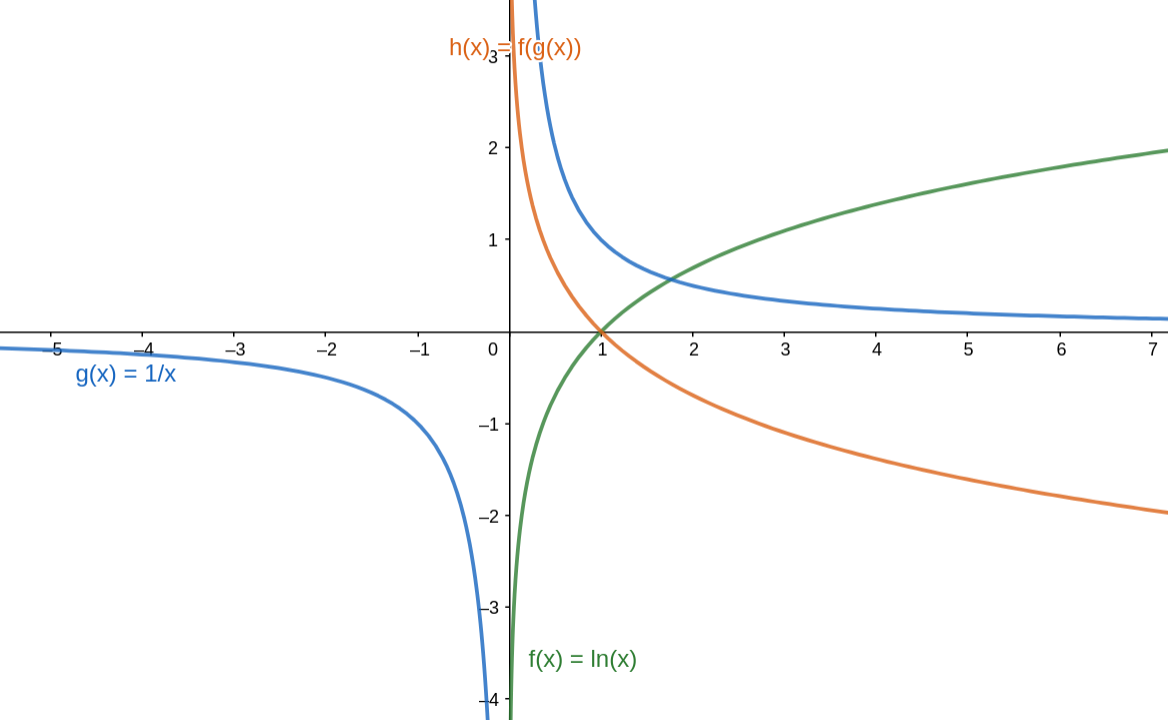换句话说，我们用对数函数，对前面的似然函数进行转换之后，是不会影响原来似然函数的变化趋势的，即对数似然函数的最大值点就是似然函数的最大值点，这个结论太重要啦！

对数似然函数的的主要作用，就是用来定义某个机器学习模型的损失函数，线性回归或者逻辑回归中都可以用到，然后我们再根据梯度下降/上升法求解损失函数的最优解，取得最优解时对应的参数θ，就是我们机器学习模型想要学习的参数 !

# 4. 总结

本文采用循序渐进的方式，一步步揭示了似然与概率之间的区别与联系，由似然我们联想到了最大似然，由最大似然我们想到了利用对数似然函数，来求解极大似然估计，希望本文能够对正在学习机器学习算法的同学们起到一定的帮助作用。对数似然
更多相关内容
• 原标题：最大似然法估计二项式分布参数前面我们学习了解了最大似然法求解正态分布参数，求解指数分布参数。今天我们再来看看最大似然法如何求解二项式分布参数。1.二项式分布与似然值估计公式二项分布基本公式求发生...

原标题：最大似然法估计二项式分布参数

前面我们学习了解了最大似然法求解正态分布参数，求解指数分布参数。今天我们再来看看最大似然法如何求解二项式分布参数。

1.二项式分布与似然值估计公式

二项分布基本公式求发生某件事情的概率：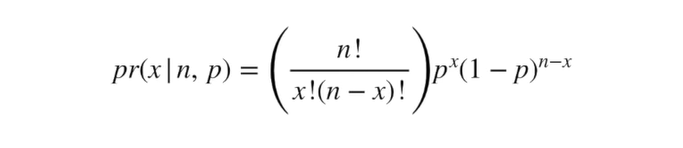如在人们对两种口味饮料无偏好时，即人们喜欢香橙口味的概率p=0.5，喜欢葡萄口味的概率p=0.5，那么7个人中4个人喜欢香橙口味的概率为0.273。计算公式如下：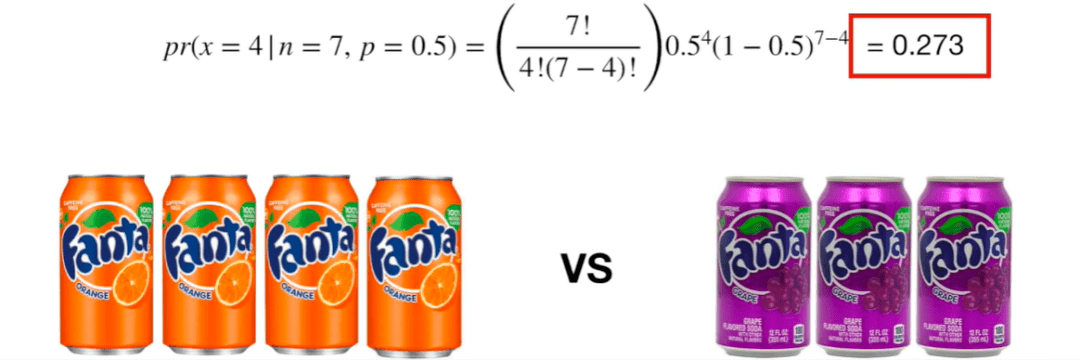似然值公式求某件事发生的环境概率：

如7个人中有4个人喜欢香橙口味饮料，在人们对两种口味饮料无偏好时，也就是喜欢两种口味饮料的概率p=0.5，那么p=0.5对应的似然值为0.273。计算公式如下：二项式分布公式与似然值公式的异同：

相同点：等式左边的写法是一样的；

不同点：

等式右边，“|”右侧的固定条件不同，也就是已知条件不同。在二项式分布公式中，固定条件为人们喜欢香橙口味的概率p=0.5,其他询问的人数。在似然值公式中，固定的条件是7个人中4个人喜欢香橙口味。“|”左边的变量不同，在二项式公式中，变量是询问人数中共有几人喜欢香橙口味；在似然值公式中，变量是人们喜欢香橙口味饮料的概率。

通过对比，能知道似然值与分布公式的重要意义： 似然值公式是通过已发生的事件，推导产生该事件环境的可能性；而 分布公式是已知环境，推导该环境下发生某件事的概率。

2.最大似然法求解二项式分布参数

「二项式分布的似然值：」用似然法估计二项式分布的参数，即我们需要计算不同p值时对应的似然值。

如下方程的含义为： 在随机7个人中4个人喜欢香橙口味的固定情况下，计算 p=0.5时的似然值为0.273；在随机7个人中4个人喜欢香橙口味的固定情况下，计算 p=0.25时的似然值为0.058；在随机7个人中4个人喜欢香橙口味的固定情况下，计算 p=0.57时的似然值为0.294。p值的取值范围是[0,1]，将以上二项式分布中的p在[0,1]范围内的似然值绘制成曲线，当曲线达到峰值(斜率为0)时对应的似然值最大。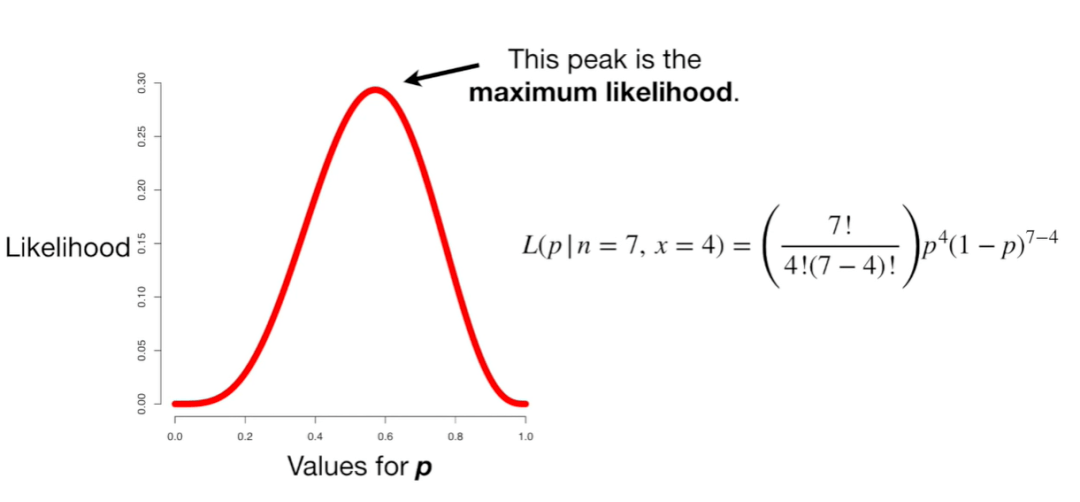「因为在似然值曲线的峰值时，该p值对应的似然值最大，故可将其转化成数学问题，求解二项式分布的导数为0时，p的取值。」

为方便求导，将似然值求解公式两边同时取对数处理并简化方程：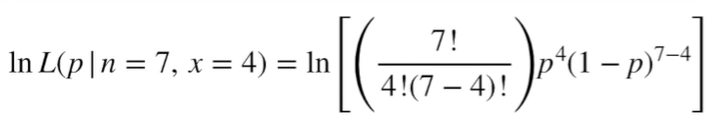函数求导并简化方程：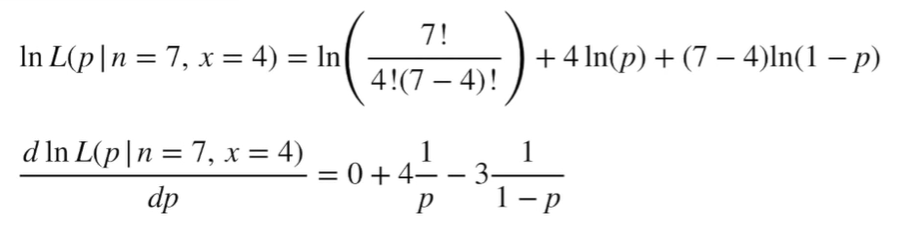令导数=0，求解p：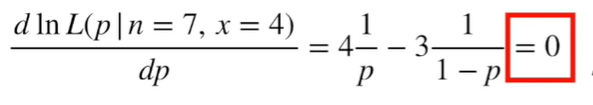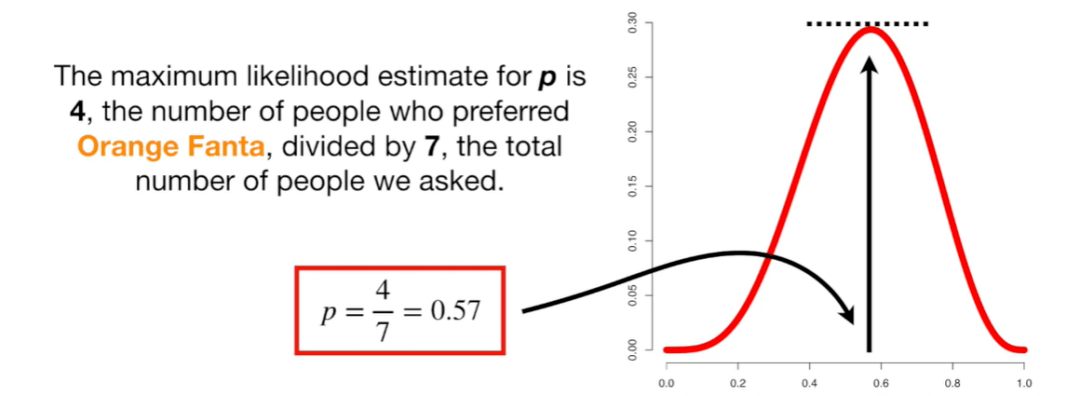当p=4/7=0.57时，取得最大似然值。故得出结论，当人们喜欢香橙口味饮料的概率为0.57时，发生4个人喜欢香橙口味，3个人喜欢葡萄口味的概率最大。

任意情况下，最大似然值估计二项式分布参数

问题：已知任意n个人中，任意x人喜欢香橙口味时，探究该二项式分布中最有可能的p值。

求解方法同前，依次对函数进行对数处理、求导、求解p。最终，得出当p=x/n时，n人中x人更喜欢香橙口味的似然值最大。

为方便求导，将似然值求解公式两边同时取对数处理并简化方程：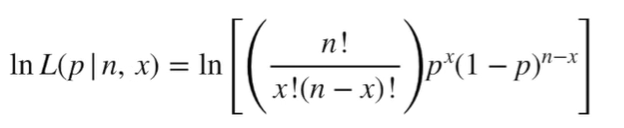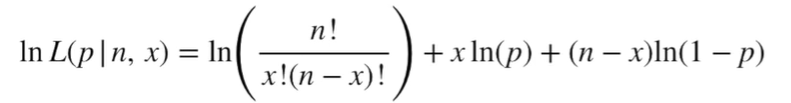因不论n与x的取值，当斜率=0(导数=0)时，该处对应的似然值最大。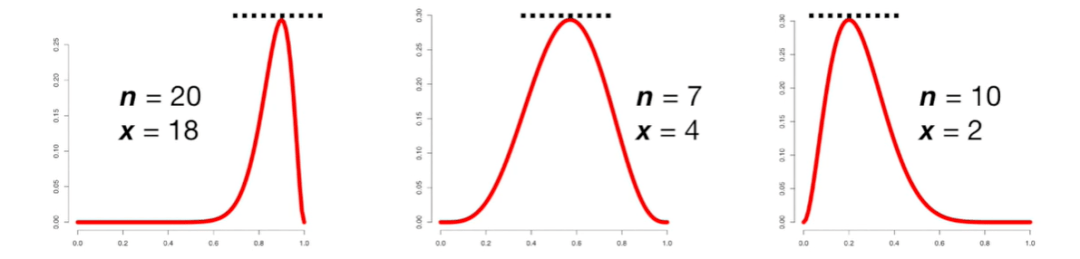令导数=0，求解p：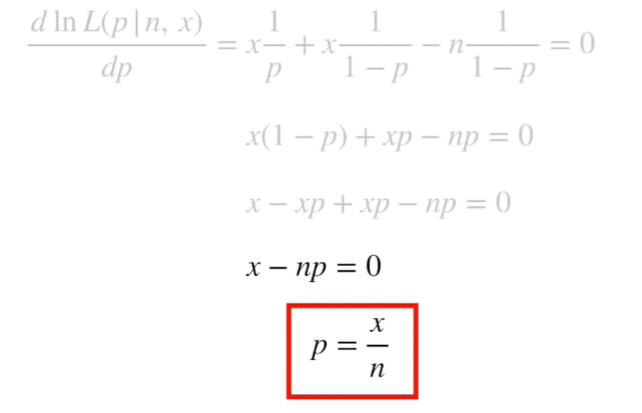得出结论：得出当p=x/n时，n人中x人更喜欢香橙口味的似然值最大，即 n人中x人更喜欢香橙口味发生的概率最大。

小结

通过前面几期的深入学习，使得我们能够更加清楚的了解最大似然值估计法的基本原理，让最大似然法不再陌生。继续加油~~~

责任编辑：

展开全文• 似然函数
似然函数在形式上，其实就是样本的联合密度。
把x1，x2，x3，．．．．．，xn看作常数，而把待定参数θ0，θ2，．．．．．，θn看作 L 的自变量。
对连续型总体X 和 离散型随机变量X，样本的似然函数分别是概率密度 和 分布率的连城形式。

极大似然估计法的基本思想：在OLS估计中，我们假定一个单一总体的参数是确定的。这个总体可以生成大量的随机样本，我们所用的样本不过是其中的一个。总之，在假设的重复抽样过程中会产生大量的样本，因而可以产生总体参数的大量样本估计值。
极大似然估计法(Maximum Likelihood Estimation，MLE)需要对随机扰动项的分布做出假定，通常选择正态分布假定。在极大似然估计中，假定样本是固定的，竹个观测值都是独立观测的，这个样本可由各种不同的总体生成，而每个样本总体都有自己的参数。那么在可供选择的总体中，哪个总体最可能生成所观测到的n个样本值? 为此需要估计每个可能总体取得这n个观测值的联合概率，选择其参数能使观测样本的联合概率最大的那个总体。

最大似然法，在二十世纪二十年代初，由费歇(R，A，Fisher l890—1962)发明的最大似然法(maximum likelihood method)是在所有估计问题中应用范围最广，并且可以从理论上证明由此得到的估计量具有几个理想的特性的方法( 见下面说明)。它可以说是统计估计理论上划时代的发现之一。设总体的概率模型为F(x|θ)。为了说明的方便，暂假定只有一个未知参数，X1，X2，……，Xn是容量为 n 的随机样本（大写X），实际观测到的样本观测值（小写x）为 Xl=x1，X2=x2，……，Xn=xn 。把同各Xi对应的密度函数或概率函数(包括作为未知数的未知参数)的连乘积看成是未知参数的函数，称其为似然函数(Likelihood function)。
也就是说，这样定义的似然函数，就是把手中得到的样本观测值实现的“概率密度或概率”，即“似然程度”看成是未知参数θ的函数。使这一似然程度为最大从而决定θ的值的“方式”，可以说是极为“合理的”估计方式。令作为样本观测值的函数被决定的θ* ＝ g(x1，x2，……，xn)对于一切可能的(先验容许的)θ值，都能满足下列条件
L(θ*)≥L(θ) ①
就是说θ*是使给定的样本观测值的似然程度为最大的θ。这时θ*叫做θ的最大似然估计值。用观测以前的样本(随机变量)X1，X2，……，Xn，代换函数g 的 n 个变量后得到的θ估计值θ^ = g(Xl，X2，……，Xn)叫做根据容量为n的样本计算的最大似然估计量。

如果所有可能的θ的集合是有限集合，要求解满足条件①式的θ值是很容易确定的，然而在大部分的应用问题中，θ的集合是无限集合。因此，在许多场合将似然函数对θ求偏导数，然后需要另外求解的方法。
此外，由于似然函数是非负的，对其进行对数变换是单调递增的变换，所以①式等价于 ㏒ L(θ*)≥㏒ L(θ)
并且， 偏导数㏒/偏导数θ = (1/L) * 偏导数L/偏导数θ
所以使logL(θ)的偏导数为0的θ值 和 使L(θ)的偏导函数为0的θ值相等。
因此，当对L(θ)直接求导比较麻烦时，可以对LogL(θ)求导，从而求得估计值θ^。

似然函数(Likelihood Function):
假定{xi}i=1→n 是从概率密度函数为f(x ; θ)的总体中抽取的独立同分布样本。目标是估计未知参数向量θ∈Rk。
似然函数定义为观察值xi的联合密度L(X；θ)，它是θ的函数：
L(x；θ) = ∏f(xi ; θ)

其中，X为样本数据矩阵，由观察值x1 , x2，……，xn组成每一行。
θ的最大似然估计量(maximum likelihood estimator，MLE)定义为θ= arg maxL(X；θ)
通常最大化对数似然函数更容易求
ζ(X；0) = Log L(X；θ)
对数似然函数与似然函数的解是等价的，因为对数转换是单调的一对一映射。即
θ = arg max L(X；θ) = argmaxf(X；θ)
最大化过程总是可以被分析表达的，即我们将得到θ估计值的显式分析表达式。然而不幸的是，在其他一些情形下，最大化过程可能是错综复杂的，牵涉到非线性最优化技术。

给定样本X和似然函数，可将运用数值方法(numerical method)来确定最大化 L(X；θ)或者ζ(X；θ)的θ值，这些数值方法通常是基于牛顿一拉普生(Newton-Raphson)迭代技术。

来自：http://www.zybang.com/question/b404a34559959d22af97c1dc3233c7ce.html

总结：似然函数与概率的区别在于，似然函数引入了参数的概念，是已知概率求参数，概率是已知参数求概率。

举例：

例子：

考虑投掷一枚硬币的实验。通常来说，已知投出的硬币正面朝上和反面朝上的概率各自是pH = 0.5，便可以知道投掷若干次后出现各种结果的可能性。比如说，投两次都是正面朝上的概率是0.25。用条件概率表示，就是：$P(\mbox{HH} \mid p_H = 0.5) = 0.5^2 = 0.25$

其中H表示正面朝上。

在统计学中，我们关心的是在已知一系列投掷的结果时，关于硬币投掷时正面朝上的可能性的信息。我们可以建立一个统计模型：假设硬币投出时会有pH 的概率正面朝上，而有1 − pH 的概率反面朝上。这时，条件概率可以改写成似然函数：$L(p_H = 0.5 \mid \mbox{HH}) = P(\mbox{HH}\mid p_H = 0.5) =0.25$

也就是说，对于取定的似然函数，在观测到两次投掷都是正面朝上时，pH = 0.5 的似然性是0.25（这并不表示当观测到两次正面朝上时pH = 0.5 的概率是0.25）。

如果考虑pH = 0.6，那么似然函数的值也会改变。$L(p_H = 0.6 \mid \mbox{HH}) = P(\mbox{HH}\mid p_H = 0.6) =0.36$

注意到似然函数的值变大了。这说明，如果参数pH 的取值变成0.6的话，结果观测到连续两次正面朝上的概率要比假设pH = 0.5时更大。也就是说，参数pH 取成0.6 要比取成0.5 更有说服力，更为“合理”。总之，似然函数的重要性不是它的具体取值，而是当参数变化时函数到底变小还是变大。对同一个似然函数，如果存在一个参数值，使得它的函数值达到最大的话，那么这个值就是最为“合理”的参数值。

在这个例子中，似然函数实际上等于：$L(p_H = \theta \mid \mbox{HH}) = P(\mbox{HH}\mid p_H = \theta) =\theta^2$， 其中$0 \le p_H \le 1$

如果取pH = 1，那么似然函数达到最大值1。也就是说，当连续观测到两次正面朝上时，假设硬币投掷时正面朝上的概率为1是最合理的。

类似地，如果观测到的是三次投掷硬币，头两次正面朝上，第三次反面朝上，那么似然函数将会是：$L(p_H = \theta \mid \mbox{HHT}) = P(\mbox{HHT}\mid p_H = \theta) =\theta^2(1 - \theta)$， 其中 T表示反面朝上，$0 \le p_H \le 1$

这时候，似然函数的最大值将会在$p_H = \frac{2}{3}$的时候取到。也就是说，当观测到三次投掷中前两次正面朝上而后一次反面朝上时，估计硬币投掷时正面朝上的概率$p_H = \frac{2}{3}$是最合理的。

来自：http://www.cnblogs.com/kevinGaoblog/archive/2012/03/29/2424346.html

展开全文• hello，大家好，上一篇分享了如何用R语言实现蒙特卡洛模拟，并用蒙特卡洛模拟计算了分布的...目录Newton法例1：求解方程组矩估计例2：设总体XXX服从二项分布B(k,p)B(k,p)B(k,p)，其中k,pk,pk,p为未知参数，X1,X2,…,

hello，大家好，上一篇分享了如何用R语言实现蒙特卡洛模拟，并用蒙特卡洛模拟计算了分布的均值和方差，今天给大家分享如何用R语言来进行矩估计和似然函数的求解。

因为在求解矩估计和似然函数时，可能会遇到非线性方程组，所以先给大家介绍一下如何用Newton法来求解非线性方程组。

本文所涉及的前两道例题来自于《R统计建模与R软件》——薛毅、陈立萍编著。

# Newton法

牛顿迭代法（Newton’s method）是牛顿在17世纪提出的一种在实数域和复数域上近似求解方程的方法。

r r f ( x ) = 0 f(x)=0 的根，选取 x 0 x_0 作为 r r 的初始近似值，过点 ( x 0 , f ( x 0 ) ) (x_0,f(x_0)) 做曲线 y = f ( x ) y=f(x) 的切线 L L L : y = f ( x 0 ) + f ′ ( x 0 ) ( x − x 0 ) L: y=f(x_0)+f'(x_0)(x-x_0) ，则 L L x x 轴的交点的横坐标 x 1 = x 0 − f ( x 0 ) f ′ ( x 0 ) x_1=x_0-\frac{f(x_0)}{f'(x_0)} ，称 x 1 x_1 r r 的一次近似值。过点 ( x 1 , f ( x 1 ) ) (x_1,f(x_1)) 做曲线 y = f ( X ) y=f(X) 的切线，并求该切线与 x x 轴交点的横坐标 x 2 = x 1 − f ( x 1 ) f ′ ( x 1 ) x_2=x_1-\frac{f(x_1)}{f'(x_1)} x 2 x_2 r r 的二次近似值。重复以上过程，得 r r 的近似值序列，其中， x n + 1 = x n − f ( x n ) f ′ ( x n ) x_{n+1}=x_n-\frac{f(x_n)}{f'(x_n)} 称为 r r n + 1 n+1 次近似值，上式称为牛顿迭代公式。

利用牛顿迭代算法的基本思路：确定迭代变量->建立迭代关系式->对迭代过程进行控制，接下来我们用一个例子来讲解。

## 例1：求解方程组

{ x 1 2 + x 2 2 − 5 = 0 ( x 1 + 1 ) x 2 − ( 3 x 1 + 1 ) = 0 \begin{cases} x_1^2+x_2^2-5=0\\ (x_1+1)x_2-(3x_1+1)=0 \end{cases}

1、确定迭代变量 x = ( x 1 , x 2 ) T x=(x_1,x_2)^T ，设定初始值 x ( 0 ) = ( 0 , 1 ) T x^{(0)}=(0,1)^T

2、建立迭代关系式：
x ( k + 1 ) = x ( k ) − [ J ( x ( k ) ) − 1 ] f ( x k ) x^{(k+1)}=x^{(k)}-[J(x^{(k)})^{-1}]f(x^{k})
其中 J ( x ) J(x) 为函数 f ( x ) f(x) 的Jacobi矩阵，即
J = ( ∂ f 1 ∂ x 1 ∂ f 1 ∂ x 2 … ∂ f 1 ∂ x n ∂ f 2 ∂ x 1 ∂ f 2 ∂ x 2 … ∂ f 2 ∂ x n ⋮ ⋮ ⋮ ∂ f n ∂ x 1 ∂ f n ∂ x 2 … ∂ f n ∂ x n ) J=\begin{pmatrix} \frac{\partial f_1}{\partial x_1} & \frac{\partial f_1}{\partial x_2} & \dots & \frac{\partial f_1}{\partial x_n}\\ \frac{\partial f_2}{\partial x_1} & \frac{\partial f_2}{\partial x_2} & \dots & \frac{\partial f_2}{\partial x_n}\\ \vdots & \vdots & & \vdots\\ \frac{\partial f_n}{\partial x_1} & \frac{\partial f_n}{\partial x_2} & \dots & \frac{\partial f_n}{\partial x_n} \end{pmatrix}
3、对迭代过程进行控制，即精度要求 ε = 1 0 − 5 \varepsilon = 10^{-5}

Newtons <- function(fun, x, ep = 1e-5, it_max = 100){
index <- 0; k <- 1
while (k <= it_max){
x1 <- x; obj <- fun(x)
x <- x - solve(obj$J, obj$f)
norm <- sqrt((x - x1) %*% (x - x1))
if (norm < ep){
index <- 1; break
}
k <- k + 1
}
obj <- fun(x)
list(root = x, it = k, index = index, FunVal = obj$f) }  funs <- function(x){ f <- c(x^2 + x^2 - 5, (x + 1)*x - (3*x + 1)) J <- matrix(c(2*x, 2*x, x-3, x+1), nrow = 2, byrow = T) list(f = f, J = J) }  Newtons(funs,c(0,1)) ##$root
##  1 2
##
## $it ##  6 ## ##$index
##  1
##
## $FunVal ##  1.598721e-14 6.217249e-15  所以方程的解为 x ∗ = ( 1 , 2 ) T x^*=(1,2)^T ，总共迭代了6次。 # 矩估计 ## 例2：设总体 X X 服从二项分布 B ( k , p ) B(k,p) ，其中 k , p k,p 为未知参数， X 1 , X 2 , … , X n X_1,X_2,\dots,X_n 是总体 X X 的一个样本，求参数 k , p k,p 的矩估计 k ^ , p ^ \hat{k},\hat{p} . 由二项分布的均值（一阶原点矩）和方差（二阶中心矩）可得方程组 { k p − X ˉ = 0 k p ( 1 − p ) − M 2 = 0 \begin{cases} kp-\bar{X}=0\\ kp(1-p)-M_2=0 \end{cases} moment_fun <- function(p){ f <- c(p*p-A1,p*p-p*p^2-M2) J <- matrix(c(p,p,p-p^2,p-2*p*p),nrow=2,byrow=T) list(f=f,J=J) }  x <- rbinom(100,20,0.7) n <- length(x) A1 <- mean(x) M2 <- (n-1)/n*var(x) p <- c(10,0.5) Newtons(moment_fun,p) ##$root
##  19.9129849  0.7221419
##
## $it ##  6 ## ##$index
##  1
##
## $FunVal ##  1.776357e-15 1.776357e-15  从结果可以看出，误差非常的小，但是也发现了一个弊端，在能用这个算法的情况下，计算往往也比较简单，所以它的效率相对较低。 接下来再给大家分享一个新的工具——uniroot函数，在遇到一些较为复杂的一元方程时可以用uniroot函数进行求解。 ## 例3：设总体密度函数如下， x 1 , … , x n x_1,\dots,x_n 是样本，试求未知参数的矩估计 p ( x ; θ ) = θ x θ − 1 , 0 < x < 1 , θ > 0. p(x;\theta)=\sqrt\theta x^{\sqrt\theta-1},0<x<1,\theta>0. 按一般做法我们需要由 E ( X ) = ∫ 0 1 x θ x θ − 1 d x E(X)=\int_0^1x\sqrt\theta x^{\sqrt\theta-1}\mathrm{d}x 推导出矩估计量 θ ^ \hat\theta ，现在我们不推导直接用uniroot求解； 因为这个密度函数是自定义的一个密度函数，因此我们需要先写一个服从该密度函数的随机数生成函数： rdensity <- function(n, theta){ obj <- function(x){ sqrt(theta)*x^(sqrt(theta)-1) } u <- c() while(length(u)<n){ x <- runif(1,0,1) y <- runif(1,0,sqrt(theta)) #sqrt(theta)是当x=1是所对应的密度函数值 if(y<=obj(x)){ u <- c(u,x) } } return(u) }  注： 这里的随机数生成采用的是随机投点的方式，取落在密度函数内的值。 x <- rdensity(100, 5) fun <- function(theta){ obj <- function(x) x*sqrt(theta)*x^(sqrt(theta)-1) integrate(obj, 0, 1)$value-mean(x)
}
uniroot(fun,c(1,10))
## $root ##  5.214032 ## ##$f.root
##  2.338409e-07
##
## $iter ##  6 ## ##$init.it
##  NA
##
## $estim.prec ##  6.103516e-05  注： integrate()为定积分函数。 从结果可以看出，准确率还是非常高的。 # 似然函数 最后，我们再在似然函数上进行下实验，以t分布为例； ## 例3：设总体 X X 服从自由度为p的t分布，其概率密度函数为 f ( t ; p ) = Γ ( p + 1 2 ) Γ ( p 2 ) 1 ( p π ) 1 2 1 ( 1 + t 2 p ) p + 1 2 , 其 中 Γ ( x ) = ∫ 0 ∞ t x − 1 e − t d t f(t;p)=\frac{\varGamma(\frac{p+1}{2})}{\varGamma(\frac{p}{2})}\frac{1}{(p\pi)^\frac12}\frac{1}{(1+\frac{t^2}{p})^{\frac{p+1}2}},其中\varGamma(x)=\int_0^\infty t^{x-1}e^{-t}\mathrm{d}t 其中 p p 为未知参数. X 1 , X 2 , … , X n X_1,X_2,\dots,X_n 是来自总体 X X 的样本，求 p p 的极大似然估计. 解：t分布的似然函数为 L ( p ; t ) = ∏ i = 1 n f ( t i ; p ) = [ Γ ( p + 1 2 ) Γ ( p 2 ) 1 ( p π ) p + 1 2 ] n 1 ∏ i = 1 n [ ( 1 + t i 2 p ) p + 1 2 ] L(p;t)=\prod_{i=1}^nf(t_i;p)=[\frac{\varGamma(\frac{p+1}{2})}{\varGamma(\frac{p}{2})}\frac{1}{(p\pi)^\frac{p+1}{2}}]^n\frac1{\prod_{i=1}^n[(1+\frac{t_i^2}p)^\frac{p+1}2]} 相应的对数似然函数为 ln ⁡ L ( p ; t ) = n ln ⁡ Γ ( p + 1 2 ) − n ln ⁡ Γ ( p 2 ) − n 2 ln ⁡ ( p π ) + p + 1 2 ∑ i = 1 n ln ⁡ ( 1 + t i 2 p ) \ln L(p;t)=n\ln\varGamma(\frac{p+1}{2})-n\ln{\varGamma(\frac{p}{2})}-\frac n2\ln(p\pi)+\frac{p+1}2\sum_{i=1}^n\ln(1+\frac{t_i^2}p) 得到对数似然方程 d d p ln ⁡ L ( p ; t ) = n 2 Γ ′ ( p + 1 2 ) Γ ( p + 1 2 ) − n 2 Γ ′ ( p 2 ) Γ ( p 2 ) − n 2 p − 1 2 ∑ i = 1 n ln ⁡ ( 1 + t i 2 p ) + p + 1 2 ∑ i = 1 n ( t i p ) 2 1 + t i 2 p = 0 \frac{\mathrm{d}}{\mathrm{d}p}\ln L(p;t)=\frac n2\frac{\varGamma'(\frac{p+1}2)}{\varGamma(\frac{p+1}2)}-\frac n2\frac{\varGamma'(\frac p2)}{\varGamma(\frac p2)}-\frac{n}{2p}-\frac12\sum_{i=1}^n\ln(1+\frac{t_i^2}p)+\frac{p+1}2\sum_{i=1}^n\frac{(\frac{t_i}{p})^2}{1+\frac{t_i^2}p}=0 其中 Γ ′ ( x ) = ∫ 0 ∞ t x − 1 ln ⁡ t e − t d t \varGamma'(x)=\int_0^\infty t^{x-1}\ln te^{-t}\mathrm{d}t n <- 100000 t <- rt(n, 6) lnL <- function(p){ (n/2)*digamma((p+1)/2)-(n/2)*digamma(p/2)-n/(2*p)-0.5*sum(log(1+t^2/p))+(p+1)/2*sum((t/p)^2/(1+t^2/p)) } uniroot(lnL,c(1,10)) ##$root
##  6.092751
##
## $f.root ##  -0.004239805 ## ##$iter
##  8
##
## $init.it ##  NA ## ##$estim.prec
##  6.103516e-05


注：

1、 d i g a m m a ( x ) = Γ ′ ( x ) Γ ( x ) digamma(x)=\frac{\varGamma'(x)}{\varGamma(x)}

2、因为方程中p有充当分母，因此给定的范围不能包含0，不然会报错。

# 获取代码

本文代码均已上传，关注公众号，回复“似然函数”，即可获得展开全文Newton法
• 概率函数 vs 似然函数 :p(x|θ) (概率函数是θ，已知，求x的概率。似然函数是x已知，求θ) 分布是p(x|θ)的总体样本中抽取到这100个样本的概率，也就是样本集X中各个样本的联合概率 最大似然估计为： 为了...
• 今天我们再来看看最大似然法如何求解二项式分布参数。1.二项式分布与似然值估计公式二项分布基本公式求发生某件事情的概率：如在人们对两种口味饮料无偏好时，即人们喜欢香橙口味的概率p=0.5，喜欢葡萄口味的概率p=...
• 对多乘积的求导往往非常复杂，但是对于多求和的求导却要简单的多，对数函数不改变原函数的单调性和极值位置，而且根据对数函数的性质可以将乘积转换为加减，这可以大大简化求导的过程： 在机器学习的公式...
• 最大熵模型中的对数似然函数的解释 最近在学习最大熵模型，看到极大似然估计这部分，没有看明白条件概率分布p(y|x)p(y|x)p(y|x)的对数似然函数。上网查了很多资料都没有一个合理的解释。基本直接给出对数似然函数的...
• 二项分布的极大似然估计 笔记来源：Maximum Likelihood for the Binomial Distribution, Clearly Explained!!! P(x∣n,p) P(x|n,p) P(x∣n,p) 计算二项分布的极大似然估计 L(p∣n,x) L(p|n,x) L(p∣n,x)
• 这篇博客主要讲解概率论和统计学中经常涉及到的，不是很好理解的一个概念——似然（Likelihood）及似然函数(Likelihood Function)，同时讲解了有关最大似然估计(Maximum Likelihood Estimation, MLE)的概念。...
• 使用最大似然法来求解线性模型（1） ...在Coursera机器学习课程中，第一篇练习就是如何使用最小均方差...本文从概率论的角度---最大化似然函数，来求解模型参数，得到线性模型。本文内容来源于：《A First Course
• (1) 线性回归方程、(2) 损失函数推导、(3) 似然函数、(4) 三种梯度下降方法 1.概念简述 线性回归是通过一个或多个自变量与因变量之间进行建模的回归分析，其特点为一个或多个称为回归系数的模型参数的线性组合。如...python 机器学习
• 文章目录1、最大似然估计的一般理论2、最大似然估计的性质3、最大似然估计推导逻辑回归的损失函数4、线性回归的损失函数5、小结 1、最大似然估计的一般理论 我们希望可以有些准则可以让我们从不同的模型中得到特定...逻辑回归 线性回归
• ebbr：R中的二项式的经验贝叶斯 执照： 经验贝叶斯收缩的方法和对数据的估计以及对成功/总数的多次观察。 这些方法在，但可以应用于多种数据类型。 安装 您可以使用从GitHub安装该软件包： devtools :: install_...R
• 文章目录似然函数与最大似然估计似然的概念似然函数最大似然估计伯努利分布伯努利分布下的最大似然估计高斯分布高斯分布下的最大似然估计信息量、熵、相对熵、交叉熵、机器学习中的交叉熵信息量熵相对熵（KL散度） ...
• 二项式做n次伯努利实验，规定了每次试验的结果只有两个，如果现在还是做n次试验，只不过每次试验的结果可以有多m个，且m个结果发生的概率互斥且和为1，则发生其中一个结果X次的概率就是多项式分布。 扔骰子是典型的...机器学习 人工智能
• 我们解决的办法是利用这些训练样本来估计问题中所涉及的先验概率和条件密度函数，并把这些估计的结果当作实际的先验概率和条件密度函数，然后再设计分类器。参数估计问题是统计学中的经典问题，并且已经有了一些...
• 贝叶斯估计、最大似然估计(MLE)、最大后验概率估计(MAP)这几个概念在机器学习和深度学习中经常碰到，读文章的时候还感觉挺明白，但独立思考时经常会傻傻分不清楚(????)，因此希望通过本文对其进行总结。2. 背景知识...
• 一、曲线拟合问题 给定 N 个输入样本及其标记，对新的样本，给出其标记的预测。...、曲线拟合的平方和损失函数 平方和误差函数是一种广泛使用的误差函数，在曲线拟合问题中，其定义如下： ...最大似然 平方损失 曲线拟合
• 极大似然估计法是求点估计的一种方法，最早由高斯提出，后来费歇尔(Fisher)在1912年重新提出。它属于数理统计的范畴。大学期间我们都学过概率论和数理统计这门课程。概率论和数理统计是互逆的过程。概率论可以看成是...
• 机器学习是要去学习一个目标函数fff或者说一个假设hhh，这个函数fff或者假设hhh可以正确分类数据或者正确拟合数据 机器学习是要去学习一个概率分布P(h∣D)P(h|D)P(h∣D)，这个概率表达式的含义是在给定数据集DDD上，...函数拟合 最大似然 神经网络
• 文章目录损失函数梯度 25天看完了吴恩达的机器学习以及《深度学习入门》和《tensorflow实战》两本书，吴恩达的学习课程只学了理论知识，另外两本书的代码自己敲了一遍，感觉过的太快，趁着跑cGAN的时间把两本书的...梯度
• 这是一个，能够让你拥有拟合最大盈利函数模型的估计方法。01什么是极大似然估计法极大似然估计是 1821 年由高斯提出，1912 年由费希尔完善的一种点估计方法。通俗来说，极大似然估计法其实源自生活的点点滴滴，比方...极大似然函数求解
• 知识点：伯努利分布、二项式分布、多项式分布、先验概率，后验概率，共轭分布、贝塔分布、贝塔-二项分布、负二项分布、狄里克雷分布，伽马函数、分布 一，伯努利分布(bernouli distribution) 又叫做0-1分布，...
• 他们都可以看着是参数分布，因为他们的函数形式都被一小部分的参数控制，比如正态分布的均值和方差，二项式分布事件发生的概率等。因此，给定一堆观测数据集（假定数据满足独立同分布），我们需要有一个
• 　如果随机试验仅有两个可能的结果，那么这两个结果可以用0和1表示，此时随机变量X将是一个0/1的变量，其分布是单个值随机变量的分布，称为伯努利分布。注意伯努利分布关注的是结果只有0和...二项分布 多项分布
• 在讲义的第一部分中，Ng首先讲解了什么叫做监督学习，其次讲了用最小二乘法求解的线性模型，用sigmod函数表示响应函数的logistics回归，接着，利用这两种模型，推出了一种应用十分广泛的指数分布族，在指数分布族的...机器学习...